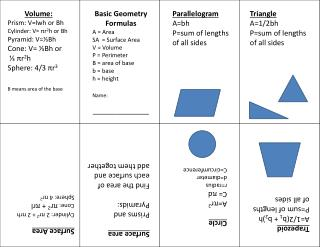DownloadDownload PresentationVolume: Prism: V= lwh or Bh Cylinder: V= π r 2 h or Bh Pyramid: V= ⅓ Bh Cone: V= ⅓ Bh or

# Volume: Prism: V= lwh or Bh Cylinder: V= π r 2 h or Bh Pyramid: V= ⅓ Bh Cone: V= ⅓ Bh or

Download Presentation## Volume: Prism: V= lwh or Bh Cylinder: V= π r 2 h or Bh Pyramid: V= ⅓ Bh Cone: V= ⅓ Bh or

- - - - - - - - - - - - - - - - - - - - - - - - - - - E N D - - - - - - - - - - - - - - - - - - - - - - - - - - -
##### Presentation Transcript

1. Volume: Prism: V=lwh or Bh Cylinder: V= πr2h or Bh Pyramid: V=⅓Bh Cone: V= ⅓Bh or ⅓ πr2h Sphere: 4/3 πr3 B means area of the base Basic Geometry Formulas A = Area SA = Surface Area V = Volume P = Perimeter B = area of base b = base h = height Name: ______________ Parallelogram A=bh P=sum of lengths of all sides Triangle A=1/2bh P=sum of lengths of all sides Surface area Prisms and Pyramids: Find the area of each surface and add them together Circle A=πr2 C= πd r=radius d=diameter C=circumference Surface Area Cylinder: 2 πr2 + 2 πrh Cone: πr2 + πrl Sphere: 4 πr2 Trapezoid A=1/2(b1 + b2)h P=sum of lengths of all sides